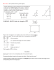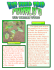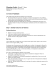# 3.3 Area and Perimeter of Parallelograms on the Coordinate Plane

## Transcription

3.3 Area and Perimeter of Parallelograms on the Coordinate Plane
```3.3 Area and Perimeter of Parallelograms on the Coordinate Plane
Bell Work:
1. What is the perimeter of the parallelogram?
2(5) + 2(4) = 18 units
5
4
3.5
2. What is the area of the parallelogram?
5(3.5) = 17.5 square units
4
5
What are the ordered pairs associated with points A, B, C, and D.
A(-3, -2)
B(-5, -5 )
C(1, -5)
D(3, -2)
Find the perimeter of the parallelogram.
Use the distance formula to find the length of each side.
Length AB =  5  3   5  2  4  9  13
Length BC = 5
2
2
Length CD = 3  1   2  5  4  9  13
2
2
A
D
B
C
Find the area of the parallelogram.
First find the height of the parallelogram. The height is the perpendicular distance between the
bases. Since the bases are horizontal the height is vertical.
Height = 3
Area = bh = 6(3) = 18 square units
The area was easy to find because the base and height were horizontal and vertical segments.
The problem becomes more difficult if the base and height are not horizontal and vertical segments.
What are the ordered pairs associated with points E, F, G, and H.
E
E(-1, 5)
F(-2, 1)
K
G(1, -3)
H(2, 1)
Find the perimeter of the parallelogram.
Use the distance formula to find the length of each side.
Length EF =
 2  12  1  52
 1  16  17
Length FG =
1  22   3  12
 9  16  5
Length GH =
2  12  1  32
 1  16  17
H
F
G
Length EH =
2  12  1  52
 9  16  5
To find the area of the parallelogram we must first find its height.
The height is the perpendicular distance between the bases. Use EH and FG as the bases.
Draw the height from F perpendicular to EH. Label the other endpoint of the height K.
Be careful, it is not so obvious where K should be or what its coordinates are.
To find the length of the height we will have to do the distance from a point to a line.
This will be a 4 step process.
a. Find the equation of the base, EH. You know the endpoints of the base so you can use these to
find the slope of EH. Then choose 1 of the two endpoints and use this and the slope in
the point slope formula, y – y1 = m(x – x1). Simplify your answer to slope-intercept form
(y = mx + b).
E(-1, 5)
H(2, 1)
m = (1 – 5) /(2 - -1) = -4/3
y – 1 = -4/3(x – 2)
y = -4/3 x + 8/3 + 1 y = -4/3 x + 11/3
b. Find the equation of the height, FK. The equation of FK is an equation of a line perpendicular to a
given line, EH, through a given point, F. This means you use the opposite reciprocal of the slope
of line EH along with the coordinates of point F in the point-slope formula: y – y1 = m(x – x1).
Since the slope of EH is -4/3 the slope of the equation of the height will be ¾ and will pass
through point F(-2, 1).
y – 1 = ¾ (x - -2)
y = ¾ x + 3/2 + 1
y = ¾ x + 5/2
c. Find the solution to the system of equations found in (a) and (b) above. This solution will be the
coordinates of point K.
y = -4/3 x + 11/3 and y = ¾ x + 5/2
-4/3 x + 11/3 = ¾ x 5/2
Multiply both sides by 12 to clear the fractions.
12(-4/3 x + 11/3) = 12(¾ x + 5/2)
-16x + 44 = 9x + 30
y = ¾(0.56) + 5/2 = 2.92
+16x – 30 +16x - 30
14 = 25x
K(0.56, 2.92)
x = 0.56
d. Use the distance formula to find the length of the height now that you know both of its
endpoints, F and K.
F(-2, 1)
K(0.56, 2.92)
0.56  22  2.92  12
 6.5536  3.6864  10.24  3.2
Area of parallelogram = bh = 5(3.2) = 16 square units
This process of finding a distance from a point to a line is a complex one.
There is an alternate approach to this problem which can also find the area of this type of
parallelogram without going through this complex process.
E
1
Surround the parallelogram with a rectangle which has sides
which are horizontal and vertical.
This creates a rectangle which is separated into 4 right triangles
and the parallelogram.
A(rectangle) = A(triangle 1) + A(triangle 2) + A(triangle 3) +
A(triangle 4) + A(parallelogram)
2
H
F
4
3
G
The area of the parallelogram can be found by finding the area
of the rectangle and each of the triangles and then
subtracting the areas of the triangles from the area of the rectangle. These areas are easy to
find because their bases and heights are all either horizontal or vertical.
Area (rectangle) = lw = 4(8) = 32
Area (triangle 1) = ½ bh = ½ (1)(4) = 2
Area (triangle 2) = ½ bh = ½ (3)(4) = 6
Area (triangle 3) = ½ bh = ½ (1)(4) = 2
Area (triangle 4) = ½ bh = ½ (3)(4) = 6
Area (parallelogram) = 32 – 2 – 6 – 2 – 6 = 16 square units
```

### Geometry Sample Questions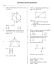### File - Mrs. Sowatsky`s Math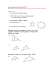### 6-3 WS 1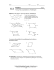### Pre-AP Geometry Worksheet Name: Section 6.3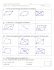### 6-3 Practice Proving That a Quadrilateral Is a Parallelogram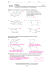### File - Waller Junior High Math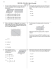### Algebra: Simplifying Algebraic Expressions, Expanding Brackets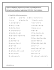### Reteaching 6-1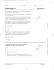### Chapter 6: Vectors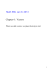### Using Both Specialisation and Generalisation in a Programming Language: Why and How?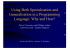### Wkst- Law of Sines-Area of Triangle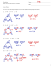### Sample Question Paper Term - II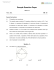### First, let’s review how to prove a parallelogram. B C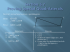### HSM12CC_GM_06_05_CM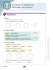### − y.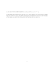### Building Shapes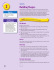### Parallelogram - Inside Mathematics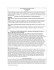### File - jsunil tutorial cbse maths & science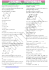### HSM12CC_GM_06_03_CM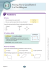### MA.7.A.1.3: Scale Factor